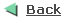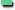How to measure Index SelectivityIndex Selectivity

B*TREE Indexes improve the performance of queries that select a small percentage of rows from a table. As a general guideline, we should create indexes on tables that are often queried for less than 15% of the table's rows. This value may be higher in situations where all data can be retrieved from an index, or where the indexed columns can be used for joining to other tables.

The ratio of the number of distinct values in the indexed column / columns to the number of records in the table represents the selectivity of an index. The ideal selectivity is 1. Such a selectivity can be reached only by unique indexes on NOT NULL columns.

Example with good Selectivity

A table having 100'000 records and one of its indexed column has 88000 distinct values, then the selectivity of this index is 88'000 / 10'0000 = 0.88.

Oracle implicitly creates indexes on the columns of all unique and primary keys that you define with integrity constraints. These indexes are the most selective and the most effective in optimizing performance. The selectivity of an index is the percentage of rows in a table having the same value for the indexed column. An index's selectivity is good if few rows have the same value.

lf an index on a table of 100'000 records had only 500 distinct values, then the index's selectivity is 500 / 100'000 = 0.005 and in this case a query which uses the limitation of such an index will retum 100'000 / 500 = 200 records for each distinct value. It is evident that a full table scan is more efficient as using such an index where much more I/O is needed to scan repeatedly the index and the table.How to Measure Index Selectivity ?

Manually measure index selectivity

The ratio of the number of distinct values to the total number of rows is the selectivity of the columns. This method is useful to estimate the selectivity of an index before creating it.

select count (distinct job) "Distinct Values" from emp;

Distinct Values
---------------
5

select count(*) "Total Number Rows" from emp;

Total Number Rows
-----------------
14

Selectivity = Distinct Values / Total Number Rows
= 5 / 14
= 0.35

Automatically measure index selectivity

We can determine the selectivity of an index by dividing the number of distinct indexed values by the number of rows in the table.

create index idx_emp_job on emp(job);
analyze table emp compute statistics;

select distinct_keys from user_indexes
where table_name = 'EMP'

and index_name = 'IDX_EMP_JOB';

DISTINCT_KEYS
-------------
5

select num_rows from user_tables
where table_name = 'EMP';

NUM_ROWS
---------
14

Selectivity = DISTINCT_KEYS / NUM_ROWS = 0.35Selectivity of each individual Column

Assuming that the table has been analyzed it is also possible to query USER_TAB_COLUMNS to investigate the selectivity of each column individually.

select column_name, num_distinct
from user_tab_columns
where table_name = 'EMP';

COLUMN_NAME                     NUM_DISTINCT
------------------------------ ------------
EMPNO                                     14
ENAME                                     14
JOB                                        5
MGR                                        2
HIREDATE                                  13
SAL                                       12
COMM                                       4
DEPTNO                                     3How to choose Composite Indexes ?

A composite index contains more than one key column. Composite indexes can provide additional advantages over single column indexes.

 Better Selectivity Sometimes two or more columns, each with poor selectivity, can be combined to form a composite index with good selectivity. Adding Data Storage If all the columns selected by the query are in the composite index, Oracle can return these values from the index without accessing the table. However in this case, it's better to use an IOT (Index Only Table).

An SQL statement can use an access path involving a composite index if the statement contains constructs that use a leading portion of the index. A leading portion of an index is a set of one or more columns that were specified first and consecutively in the list of columns in the CREATE INDEX statement that created the index. Consider this CREATE INDEX statement:

CREATE INDEX idx_composite ON mytab (x, y, z);

These combinations of columns are leading portions of the index: X, XY, and XYZ. These combinations of columns are not leading portions of the index: YZ and Z.Guidelines for choosing columns for composite indexes

Consider creating a composite index on columns that are frequently used together in WHERE clause conditions combined with AND operators, especially if their combined selectivity is better than the selectivity of either column individually. Consider indexing columns that are used frequently to join tables in SQL statements.